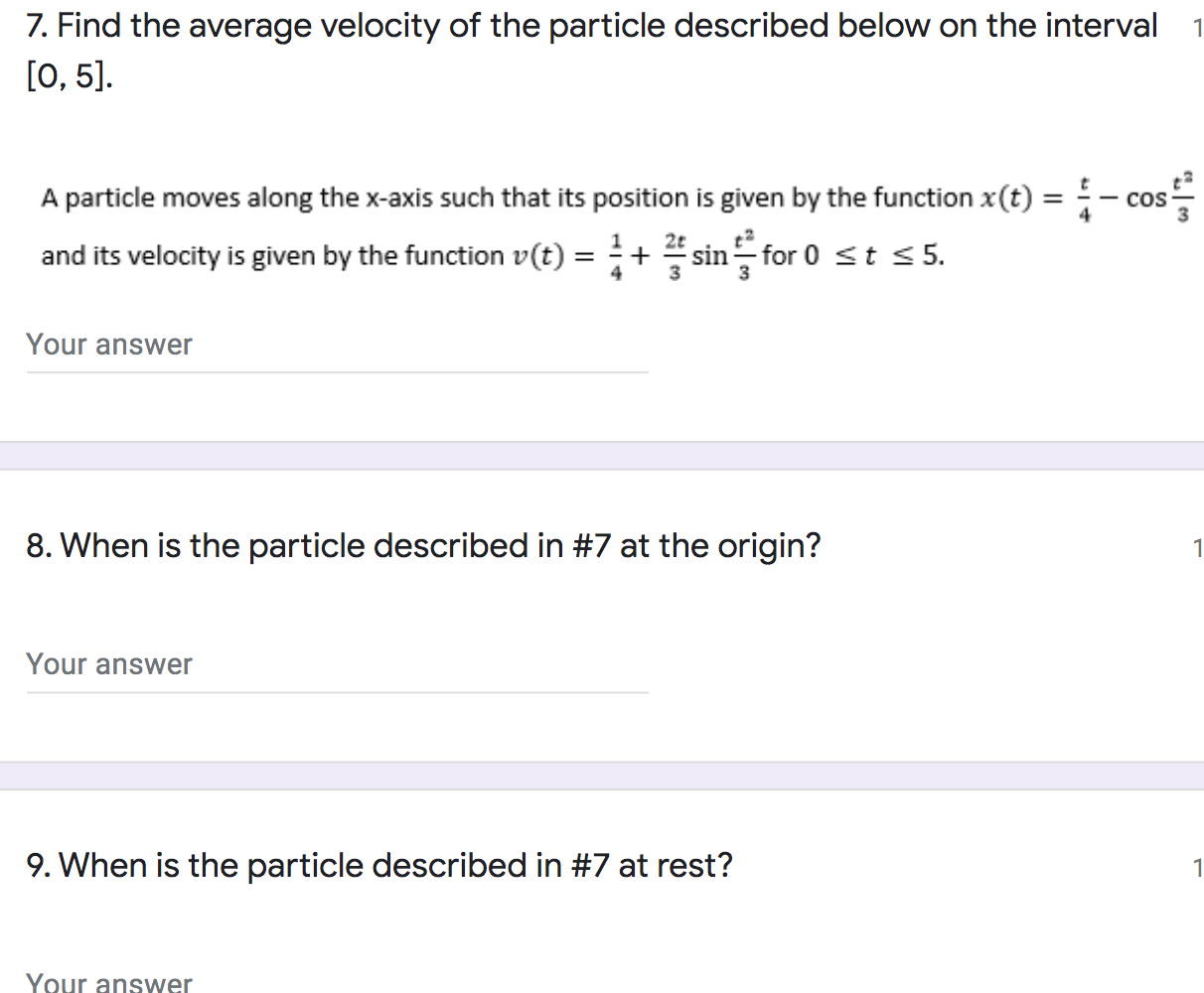### Create an Account

Home / Questions / 1 7. Find the average velocity of the particle described below on the interval [0, 5]. A p...

# 1 7. Find the average velocity of the particle described below on the interval [0, 5]. A particle moves along the x-axis such that its position is given by the function x(t) = and its velocity is give

1 7. Find the average velocity of the particle described below on the interval [0, 5]. A particle moves along the x-axis such that its position is given by the function x(t) = and its velocity is given by the function v(t) for 0 st 55. - cos Your answer 8. When is the particle described in #7 at the origin? 1 Your answer 9. When is the particle described in #7 at rest? 1 Your answer
11. At t = 3, is the speed of the particle in #7 increasing or decreasing? (one word answer)May 14 2021 View more View LessSubscribe To Get Solution